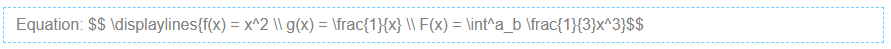# Equations with LaTeX

Mathematical equations may be created using LaTeX notation.

While editing a chapter, select Insert > LaTeX Equation (Block) . In the resulting box, type your equation between the double-dollar signs.

You can continue to edit the formula in the editor (provided that you do not remove the double-\$ symbols). When saved, the example equation will render like this:

$$E=mc^2$$

Here is a more sophisticated example:

Which will render like this:

$$S (\omega)=1.466\, H_s^2 \, \frac{\omega_0^5}{\omega^6 } \, e^[-3^ { \omega/(\omega_0 )]^2}$$

## Inline Equations

Equations may be typed directly into text, such as $$E=mc^2$$, by placing them within slash parentheses. You can also insert an inline equation by right-clicking and choosing Insert > LaTeX Equation (Inline).$$\displaylines{f(x) = x^2 \\ g(x) = \frac{1}{x} \\ F(x) = \int^a_b \frac{1}{3}x^3}$$